#FutureSTEMLeaders - Wiingy's \$1200 scholarship for School and College Students

Apply Now

AP Statistics

# Departure from Linearity Meaning

Written by Prerit Jain### Departure from Linearity Meaning

According to the linearity assumption, the combined effects of several variables, whether transformed or untransformed, result in a model with residuals that are regularly and independently distributed at random.

One of the most important statistical models is linear regression which is used widely for the analysis of data. The linear regression technique assumes that the parameters of the independent variables and the dependent variable Y have a linear relationship. We cannot apply the model if the real connection is not linear since the accuracy will be drastically decreased.

## Types of departures from linearity

In statistics, the word “nonlinearity” is used to describe a scenario in which an independent variable and a dependent variable do not have a straight-line or direct connection. Changes in the output are not directly proportional to changes in any of the inputs in a nonlinear connection.

• Nonlinearity in the predictor variable

The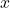variable is known as the predictor variable or the independent variable. The outlier in the x-axis shows the nonlinearity.

• Nonlinearity in the response variable

The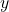variable is known as the response variable. A point that is an outlier in the y-direction alone obviously doesn’t fit the data. However, removing it won’t make a significant impact on the slope because it doesn’t have a lot of leverage. The slope is controlled by the many other points that do fit the pattern. This is the nonlinearity in the response variable.

• Interaction effects

When the values of one independent variable affect how a result is affected by another independent variable, this is known as an interaction. The interaction effects are interpreted as follows:

1. If the lines are not parallel there is an interaction.

2. If the lines are parallel there is an interaction.

• Non-constant variance

When there is non-constant variance there is a term called Heteroskedasticity

that occurs. When the error term or the residual variance’s variance varies across observations, heteroskedasticity is present. On a graph, this indicates that there are a variety of points around the regression line.

## Identifying departures from linearity

• Visualizing linearity through scatter plots and residual plots

In a scatterplot, a linear relationship may be shown. This indicates that the scatterplot’s points closely approximate a straight line. If one variable rises roughly at the same pace as the other variables change by one unit, the connection is said to be linear.

A residual plot demonstrates how the data points stray from the model as a residual is the “leftover” value after subtracting the expected value from the actual value and the expected value is determined by a linear model, such as a line of best fit.

If the residuals are evenly distributed around the residual = 0, then a linear model accurately represents the data points without preferring any particular inputs. We come to the conclusion that a linear model is adequate in this scenario.

• Formal tests for linearity, such as the Breusch-Pagan test and the White test

1. Breusch-Pagan test: To ascertain whether heteroscedasticity exists in a regression model we apply the Breusch-Pagan test. We first set the hypotheses and then conduct the test. The steps for the test are as follows:

• Find the regression model
• Find the squared residuals for the model
• Fit a new model with the calculated residuals
• Find the Chi-Square statistic.

If the calculated p-value is less than a significance level of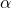then reject the hypotheses, else accept it.

2. White test: This test is the same as the Breusch-Pagan test but the only difference is that its auxiliary regression doesn’t include cross-terms or the original squared variables.

## Handling departures from linearity

• We can transfer the variables to achieve linearity by using,
1. Powers and roots
2. Logarithms.

Depending on the relation between the variables.

• Nonlinear regression models, such as polynomial and spline regression
1. Polynomial regression: The straightforward method to model non-linear relationships is this one. To a regression, it adds polynomial terms or quadratic terms.
2. Spline regression: Splines offer a method for smoothly interpolating between knots or fixed points. Between knots, polynomial regression is calculated. Splines are just a string of knotted polynomial segments that have been tied together.
• Robust regression methods: Iteratively reweighted least squares is a technique used in robust regression to give each data point a weight. Large changes in minor portions of the data will have less of an impact using this strategy. Therefore, compared to conventional linear regression, robust linear regression is less susceptible to outliers.
• Mixed-effects models: The last illustration provides a direct transition into a mixed-effect model. In this model, we may keep some low-level components while allowing some information from the overall regression to be included in the low-level regressions.

## R squared intuition

The quality of fit of a model is gauged by thevalue. A statistical indicator of how well the regression predictions match the actual data points in a regression is thecoefficient of determination. A regression forecast that completely matches the data has anof 1.

• Interpreting Computer regression data

The steps to interpret computer output for regressions are as follows:

1. Insert the x-values in the first column and the y-values in the second column given the data points, …,.
2. To find the equation of the linear regression lineand the correlation coefficient r, use the columns from Step 1.
3. To get the residuals’ standard deviation, use the columns from Step 1.

Outliers can cause the R-Squared statistic to overstate or understate the main trend in the data, respectively. Therefore, the removal of outliers will help to analyze the statistics in the right manner.

## Conclusion

In this article, we learned about why linearity is important in Statistics and what are the different kinds of departures from linearity and how to identify and fix them. We see the non-linearity in a lot of cases of real-life applications, which is undesirable. We learned how to use tests to identify them and how to handle them.

## Sample examples

Example 1: A transformed data model has the following equation:. To transform the data back to the original we have to use the formula. Find the values of a and b.

Solution 1:

We use converting the equation given to fit the equation desired.

Taking both sides to the power of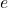we get,Therefore, the values of a and b are 0.5 and 2 respectively.

Example 2: A data model has the equation. Convert this equation to handle the departure from linearity.

Solution 2:

We use converting the equation given to fit the equation desired.

Taking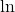on both sides we get fit with lesser outliers.

Therefore, the equation would beExample 3: A model has the equation. Convert this equation to handle the departure from linearity.

Solution 3:

We use converting the equation given to fit the equation desired.### What is linear regression?

A variable’s value can be predicted using linear regression analysis based on the value of another variable. The dependent variable is the one you want to be able to forecast. The independent variable is the one you’re using to make a prediction about the value of the other variable.

### What is a variable?

Any traits, figures, or amounts that may be measured or counted are considered variables.

### What is a spline?

Splines combine curves to create continuous, asymmetrical shapes.

### What is Chi-square?

A chi-squared test is essentially a data analysis based on observations of a random set of variables. Typically, it involves a contrast between two sets of statistical data. curves.

### What is heteroscedasticity?

When a predicted variable’s standard deviations are not constant across time or for changing values of an independent variable, this is known as heteroscedasticity in statistics.

Written by

Prerit Jain